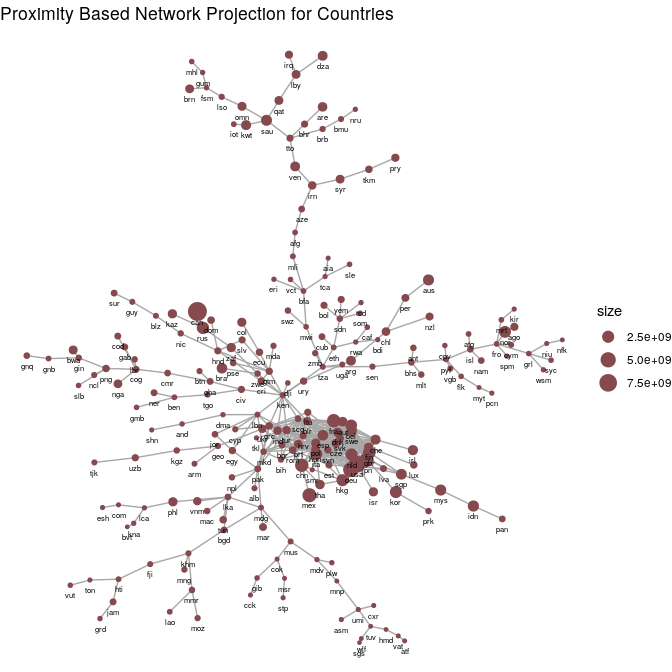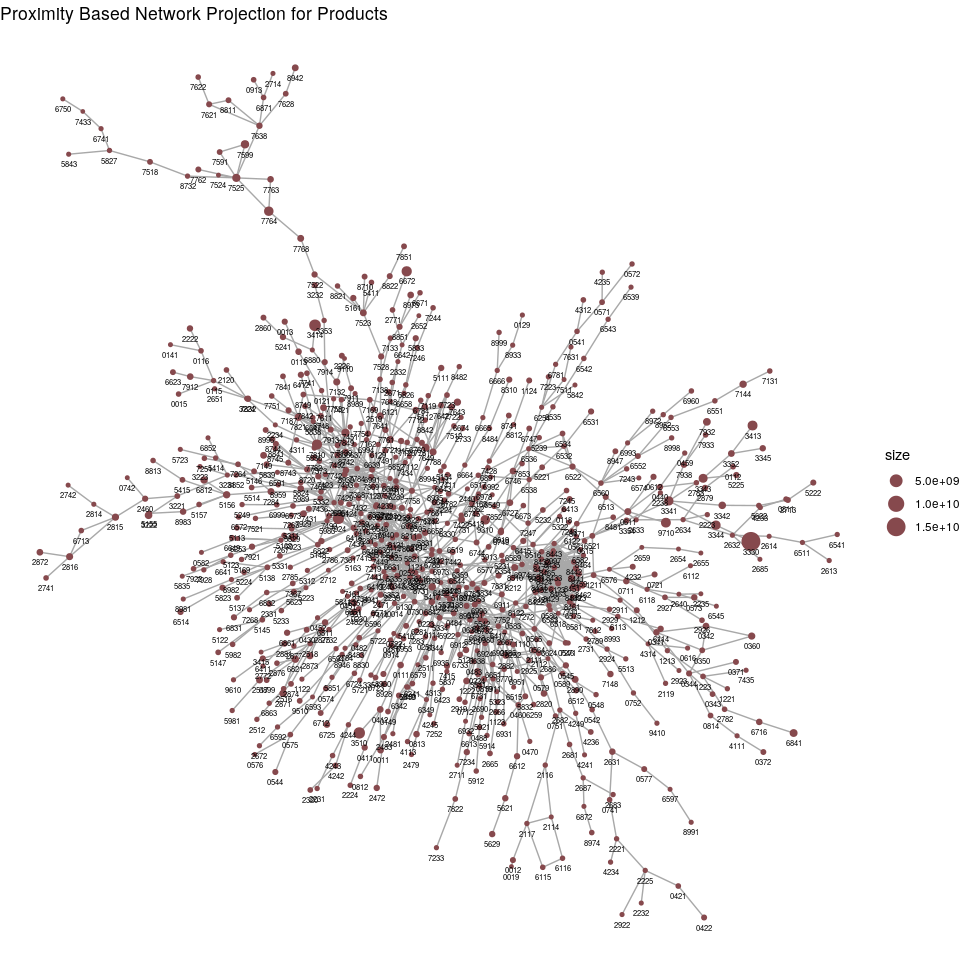# World Trade and Per-Capita GDP

This example is inspired after the visualizations from Hausmann et al. (2014) with some ggplot additions.

## Package Data

library(economiccomplexity)

# partial view of trade matrix
head(world_trade_avg_1998_to_2000)
##   country product value
## 1     afg    0011 30068
## 2     afg    0012 16366
## 3     afg    0111 19273
## 4     afg    0112   893
## 5     afg    0113   350
## 6     afg    0116  1561
# partial view of gdp vector
head(world_gdp_avg_1998_to_2000)
##   country value
## 1     abw 19185
## 2     ago  1540
## 3     alb  1433
## 4     and 27765
## 5     arb  3312
## 6     are 43082

## Balassa Index

You can obtain Balassa Index with balassa_index().

bi <- balassa_index(world_trade_avg_1998_to_2000)

# partial view of index
head(bi)
##  0 0 0 0 1 0

Another possibility is to obtain Balassa Index without discretization.

bi_dec <- balassa_index(world_trade_avg_1998_to_2000, discrete = F)

# partial view of index
head(bi_dec)
##  0.2312238 0.1777917 0.0000000 0.0000000 2.4825815 0.0000000

## Complexity Measures

You can compute complexity indexes (e.g. such as the Economic Complexity Index and Product Complexity Index) by using complexity_measures(). The calculations methods are fitness (default), reflections, eigenvalues. See (Mariani et al. 2015) for the methodological details.

The eigenvalues also calls the reflections methods in order to correct the index sign in some special cases when the correlation between the output from both methods is negative.

### Complexity-Fitness

com_fit <- complexity_measures(bi)

# partial view of indexes
com_fit$complexity_index_country[1:5] ## afg ago aia alb and ## 0.78605655 0.03999516 1.05645538 1.24261128 1.37321261 com_fit$complexity_index_product[1:5]
##      0011      0012      0013      0014      0015
## 0.7538883 0.7491391 2.3018690 0.9903918 1.3120378

### Reflections

com_ref <- complexity_measures(bi, method = "reflections")

# partial view of indexes
com_ref$complexity_index_country[1:5] ## afg ago aia alb and ## -0.5788151 -1.7710696 1.4074821 -0.1754989 1.0738736 com_ref$complexity_index_product[1:5]
##        0011        0012        0013        0014        0015
## -0.66255107 -1.62169899 -0.07449487  0.20554720  0.15848845

### Eigenvalues

com_eig <- complexity_measures(bi, method = "eigenvalues")

# partial view of indexes
com_eig$complexity_index_country[1:5] ## afg ago aia alb and ## -0.5764283 -1.7770752 1.4090414 -0.1732606 1.0772452 com_eig$complexity_index_product[1:5]
##        0011        0012        0013        0014        0015
## -0.66657613 -1.62657599 -0.08149436  0.19917280  0.14935653

## Proximity

Proximity matrices are used to create projections e.g. (country-country and product-product networks) for bipartite networks. Using proximity() is straightforward.

pro <- proximity(bi)

# partial view of proximity matrices
pro$proximity_country[1:5,1:5] ## 5 x 5 sparse Matrix of class "dsCMatrix" ## afg ago aia alb and ## afg 1.00000000 0.015873016 0.181818182 0.19689119 0.192513369 ## ago 0.01587302 1.000000000 0.006993007 0.01554404 0.005347594 ## aia 0.18181818 0.006993007 1.000000000 0.16580311 0.251336898 ## alb 0.19689119 0.015544041 0.165803109 1.00000000 0.310880829 ## and 0.19251337 0.005347594 0.251336898 0.31088083 1.000000000 pro$proximity_product[1:5,1:5]
## 5 x 5 sparse Matrix of class "dsCMatrix"
##           0011      0012      0013      0014      0015
## 0011 1.0000000 0.3658537 0.1707317 0.2439024 0.2682927
## 0012 0.3658537 1.0000000 0.2500000 0.2250000 0.2500000
## 0013 0.1707317 0.2500000 1.0000000 0.2500000 0.1200000
## 0014 0.2439024 0.2250000 0.2500000 1.0000000 0.2250000
## 0015 0.2682927 0.2500000 0.1200000 0.2250000 1.0000000

## Projections

The projections() function is designed to use igraph for the internal computations and also to pass proximity-based networks to igraph, ggraph or export to Cytoscape by saving the output as csv/tsv.

library(igraph)

net <- projections(pro$proximity_country, pro$proximity_product)

# partial view of projections
E(net$network_country)[1:5] ## + 5/484 edges from c5f4181 (vertex names): ##  zaf--zwe tza--zmb tza--uga tuv--wlf tuv--umi E(net$network_product)[1:5]
## + 5/1505 edges from f4cb75f (vertex names):
##  8981--8982 8946--9510 8922--8932 8921--8922 8852--8959

Just two basic examples with ggraph.

set.seed(200100)

library(Matrix)
library(ggraph)

aggregated_countries <- aggregate(
world_trade_avg_1998_to_2000$value, by = list(country = world_trade_avg_1998_to_2000$country),
FUN = sum
)

aggregated_countries <- setNames(aggregated_countries$x, aggregated_countries$country)

V(net$network_country)$size <- aggregated_countries[match(V(net$network_country)$name, names(aggregated_countries))]

ggraph(net$network_country, layout = "kk") + # geom_edge_link(aes(edge_width = weight), edge_colour = "#a8a8a8") + geom_edge_link(edge_colour = "#a8a8a8") + geom_node_point(aes(size = size), color = "#86494d") + geom_node_text(aes(label = name), size = 2, vjust = 2.2) + ggtitle("Proximity Based Network Projection for Countries") + theme_void()set.seed(200100) aggregated_products <- aggregate( world_trade_avg_1998_to_2000$value,
by = list(country = world_trade_avg_1998_to_2000$product), FUN = sum ) aggregated_products <- setNames(aggregated_products$x, aggregated_products$country) V(net$network_product)$size <- aggregated_products[match(V(net$network_product)$name, names(aggregated_products))] ggraph(net$network_product, layout = "kk") +
# geom_edge_link(aes(edge_width = weight), edge_colour = "#a8a8a8") +
geom_node_point(aes(size = size), color = "#86494d") +
geom_node_text(aes(label = name), size = 2, vjust = 2.2) +
ggtitle("Proximity Based Network Projection for Products") +
theme_void()# Complexity Outlook

Both the Complexity Outlook Index and Complexity Outlook Gain are obtained after the complexity_outlook() function.

co <- complexity_outlook(
economiccomplexity_output$balassa_index, economiccomplexity_output$proximity$proximity_product, economiccomplexity_output$complexity_measures$complexity_index_product ) # partial view of complexity outlook co$complexity_outlook_index[1:5]
##        afg        ago        aia        alb        and
## 103.948610   9.962401 122.311158 152.107317 151.295380
co$complexity_outlook_gain[1:5,1:5] ## 5 x 5 Matrix of class "dgeMatrix" ## 0011 0012 0013 0014 0015 ## afg 0.8615531 0.7613878 0.7537907 1.0961458 0.8143851 ## ago 0.9681802 0.8436855 0.8219918 1.2151040 0.8883146 ## aia 0.8339114 0.7475080 0.7247198 1.0425043 0.7671877 ## alb 0.7979779 0.7199705 0.7132042 1.0170093 0.7636545 ## and 0.0000000 0.7118512 0.6829531 0.9987236 0.0000000 # Productivy Levels The productivity_levels() dataset follows the definitions from Hausmann et al. (2014) and Hausmann, Hwang, and Rodrik (2005). I don’t have a per-capita GDP dataset for the Galactic Federation, so I’ll create simulated data for the example. pl <- productivity_levels(world_trade_avg_1998_to_2000, world_gdp_avg_1998_to_2000) # partial view of productivity levels pl$productivity_level_country[1:5]
##       ago       alb       and       are       arg
##  8223.607  6343.341 13783.485 10207.679  9269.670
pl\$productivity_level_product[1:5]
##      0011      0012      0013      0014      0015
##  7915.893  3986.371 11375.710  6273.428 17628.950